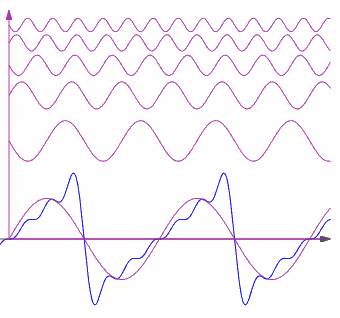Search IntMath
Close

450+ Math Lessons written by Math Professors and Teachers

5 Million+ Students Helped Each Year

1200+ Articles Written by Math Educators and Enthusiasts

Simplifying and Teaching Math for Over 23 Years

# Fourier Series interactive graph

By Murray Bourne, 17 Dec 2012

I recently developed an interactive Fourier Series graph that demonstrates how the series works.

Fourier Series are used in various branches of engineering to solve problems involving heat, acoustics, image processing and optics.

The basic idea of a Fourier Series is that we can decompose a periodic function into the sum of simpler sine and cosine curves. This was quite a revolutionary idea when French mathematician Jean-Baptiste Joseph Fourier proposed it as a solution for the heat equation in the early 19th century. His practical problem involved determining how heat is distributed through a metal plate.Still image from interactive:
Fourier Series terms (in pink) and resulting sum (in blue)

We obtain the terms in the Fourier Series using integration. You can see the processes involved and some examples on this page: Full Range Fourier Series.

The interactive graph shows how the individual terms in the series (the sine or cosine graphs) and the result of adding those terms to some fundamental constant value.

The interactive includes 3 different Fourier Series examples.

## Geek info

I used JXSGraph to draw the graph, MathJax for the equations, and stitched it together using jQuery and other javascript.

The link again: Interactive Fourier Series graph

### 2 Comments on “Fourier Series interactive graph”

1. april says:

What did you used to graph this?? I did try it in scilab, but unfortunately i wasn't able to make a code.

2. Murray says:

@April - I have amended the post so it now includes a mention of the technologies involved. Hope it helps.

### Comment Preview

HTML: You can use simple tags like <b>, <a href="...">, etc.

To enter math, you can can either:

1. Use simple calculator-like input in the following format (surround your math in backticks, or qq on tablet or phone):
a^2 = sqrt(b^2 + c^2)
(See more on ASCIIMath syntax); or
2. Use simple LaTeX in the following format. Surround your math with $$ and $$.
$$\int g dx = \sqrt{\frac{a}{b}}$$
(This is standard simple LaTeX.)

NOTE: You can mix both types of math entry in your comment.